Chapter 2: Inverse Trigonometric Functions

## Chapter 2: Inverse Trigonometric Functions

#### Function

If y = f(x) and x = g(y) are two functions such that f (g(y)) = y and g (f(y)) = x, then f and y are said to be inverse of each other
i.e., g = f-1 IF y = f(x), then x = f-1(y)

#### Inverse Trigonometric Functions

If y = sin X-1, then x = sin-1 y, similarly for other trigonometric functions.
This is called inverse trigonometric function .
Now, y = sin-1(x), y ∈ [π / 2 , π / 2] and x ∈ [-1,1].
(i) Thus, sin-1x has infinitely many values for given x ∈ [-1, 1].
(ii) There is only one value among these values which lies in the interval [π / 2 , π / 2]. This value is called the principal value.

##### Domain and Range of Inverse Trigonometric FunctionsGraphs of Inverse Trigonometric Functions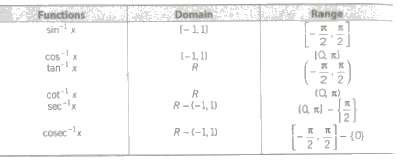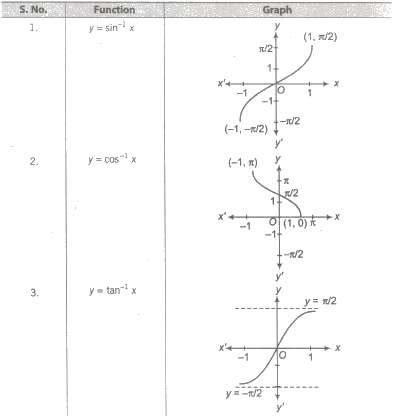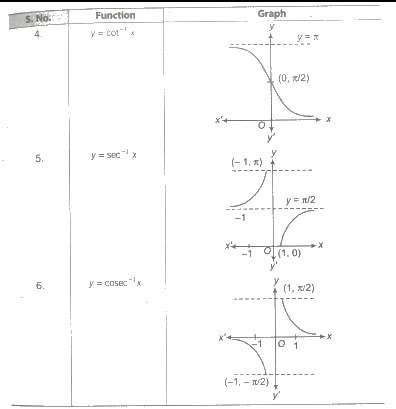#### Properties of Inverse Trigonometric Functions

##### Property I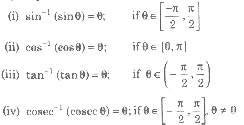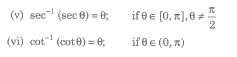##### Property II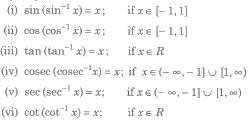##### Property III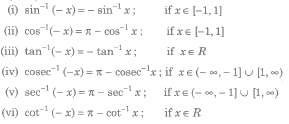##### Property IV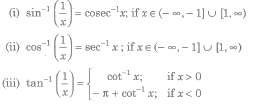##### Property V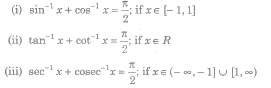##### Property VI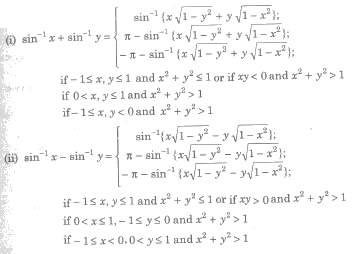###### Property VII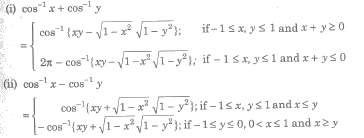###### Property VIII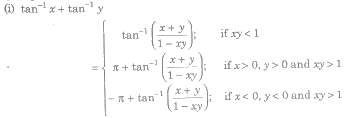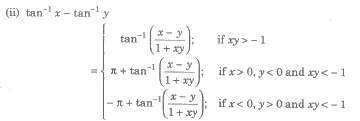###### Property IX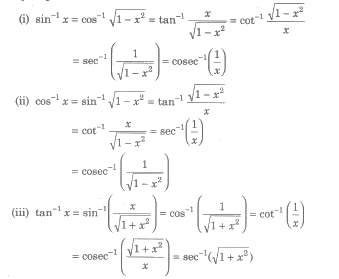###### Property X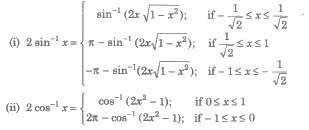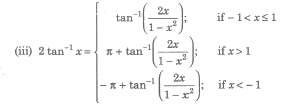###### Property XI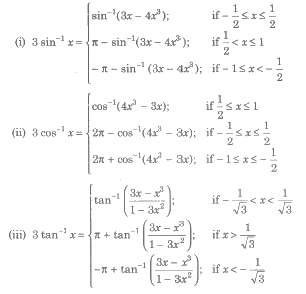###### Property XII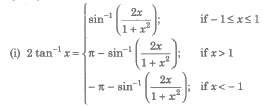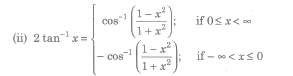#### Important Results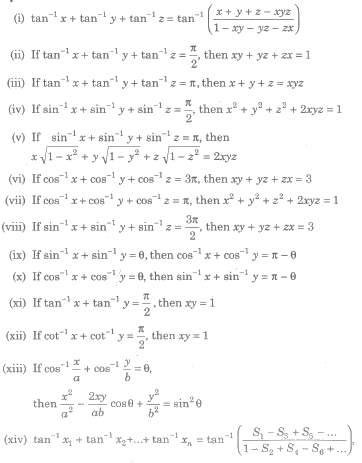where Sk denotes the sum of the product of x1,x2,…xn takes k at a time.

#### Inverse

##### Trigonometric Equation
An equation involving one or more trigonometrical ratios of unknown angle is called a trigonometric equation .
##### Solution/Roots of a Trigonometric Equation

A value of the unknown angle which satisfies the given equation, is called a solution or root of the equation.
The trigonometric equation may have infinite number of solutions.
(i) Principal Solution – The least value of unknown angle which satisfies the given equation, is called a principal solution of trigonometric equation.
(ii) General Solution – We know that, trigonometric function are periodic and solution of trigonometric equations can be generalised with the help of the periodicity of the trigonometric functions.
The solution consisting of all possible solutions of a trigonometric equation is called its general solution.

##### Important Results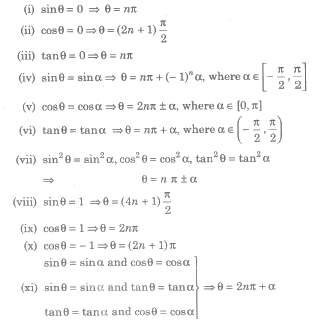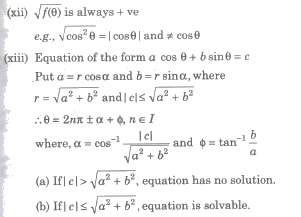##### Important Points to be Remembered

(i) While solving an equation, we have to square it, sometimes the resulting roots does not satisfy the original equation.
(ii) Do not cancel common factors involving the unknown angle on LHS and RHS.Because it may be the solution of given equation.
(iii) (a) Equation involving sec θ or tan θ can never be a solution of the form (2n + 1) π / 2.
(b) Equation involving coseca or cote can never be a solution of the form θ = nπ.

### NCERT Books Free Pdf Download for Class 5, 6, 7, 8, 9, 10 , 11, 12 Hindi and English Medium

 Mathematics Biology Psychology Chemistry English Economics Sociology Hindi Business Studies Geography Science Political Science Statistics Physics Accountancy